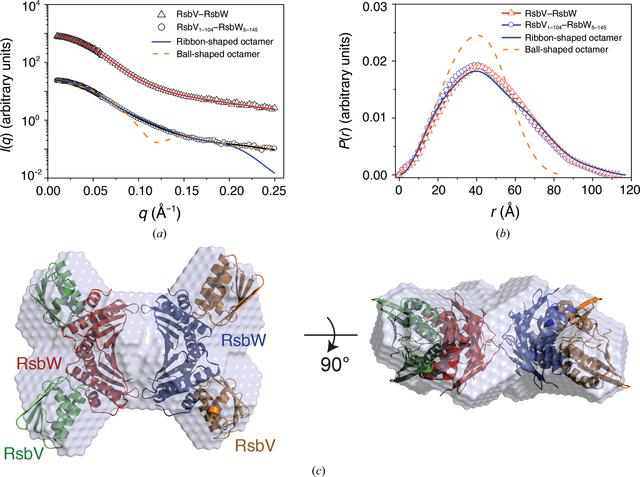disable zoom     view article Figure 6 SAXS envelope structure of the RsbV–RsbW octamer. (a) X-ray scattering profiles of full-length RsbV–RsbW (triangles) and RsbV1–104–RsbW5–145 (circles). Red and black solid lines represent SAXS curves calculated from the SAXS envelope structures (χ2 = 0.077 for full-length RsbV–RsbW and 0.179 for RsbV1–104–RsbW5–145). The solid blue and dashed orange lines indicate theoretical SAXS curves calculated from ribbon-shaped and ball-shaped crystal structures, respectively (χ2 = 8.311 for the ball-shaped model and 0.812 for the ribbon-shaped model). For clarity, each curve is shifted along the log I(q) axis. (b) p(r) function profiles of full-length RsbV–RsbW (red triangles) and RsbV1–104–RsbW5–145 (blue circles). The solid blue and dashed orange lines indicate the theoretical p(r) function calculated from the ribbon-shaped and ball-shaped octamer models, respectively. The area under the curve was normalized to an equal area for easy comparison. (c) SAXS envelope structure of RsbV1–104–RsbW5–145. The SAXS envelope model was reconstructed under a P22 symmetry restraint and is superimposed with the ribbon-shaped crystal structure of the RsbV–RsbW octamer.

IUCrJ
Volume 7| Part 4| July 2020| Pages 737-747
ISSN: 2052-2525